# SQL Windows FunctionIntroduction & Overview Types of Window Function in SQL. Explanation with an example using the PostgreSQL. A window function performs a Data Analysis calculation across a set of table rows that are somehow related to the current row. Address the comparable type of calculation can be done with an aggregate function that gives a single row or grouped by condition

Introduction & Overview Types of Window Function in SQL.

Introduction:

A window function performs a Data Analysis calculation across a set of table rows that are somehow related to the current row. Address the comparable type of calculation can be done with an aggregate function that gives a single row or grouped by condition (refer to Figure 1).

Window function does not cause rows to become grouped into a single output row. Rows retain their separate identities also able to access more than just the current row of the query result. (refer to Figure 1)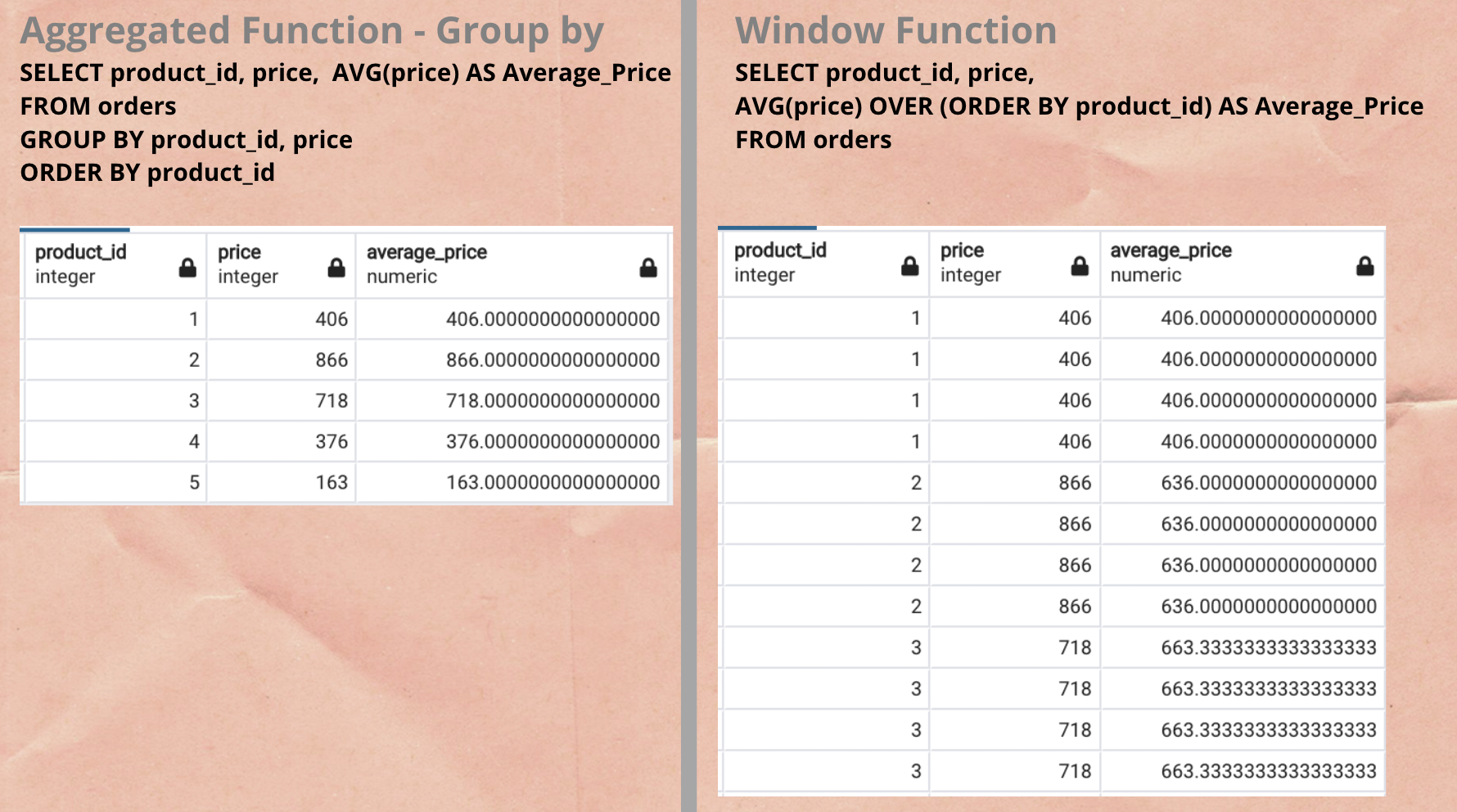Figure 1 — Difference between — Aggregated and Windows function

_The database used to explain below concepts: Postgres database and Dataset: Available at [Github Order_Table.csv_](https://github.com/GDhasade/Medium.com_Contents/tree/master/SQL)

Window Function Syntax:

``````Window_Function([All] expression)
OVER( [PARTITION BY expression_list] [ORDER BY order_list Row_or_ Range clause] )``````

Each part of syntax has been explained as follows:

Window_Function:

There are 3 major types of window_functions

1. Window Aggregated Function: Consist one of the supporting aggregated function i.e. AVG(), COUNT(), MIN(), MAX(), SUM().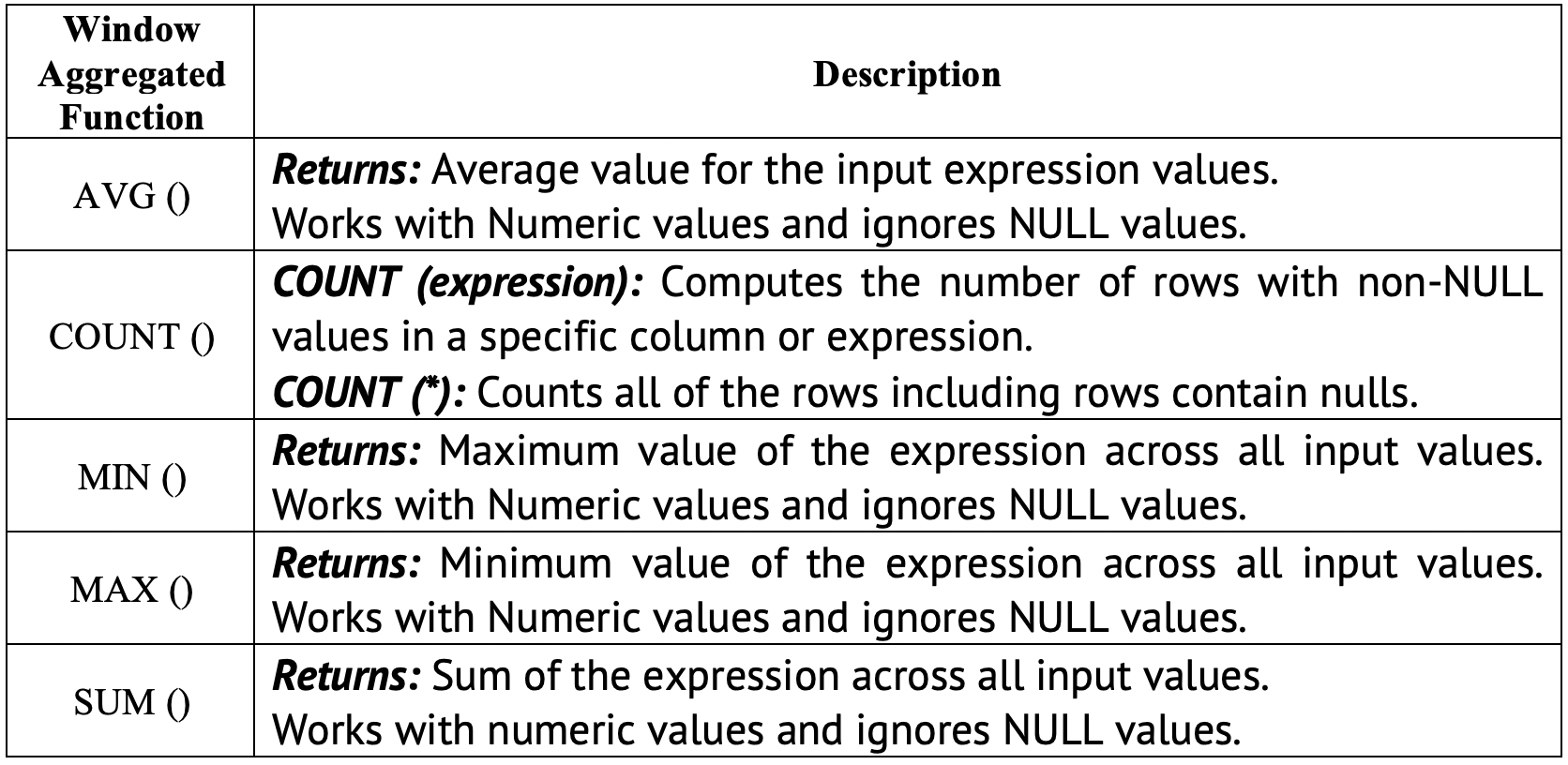Window Aggregated Function

*2. Window Ranking Aggregated Function: *Consist one of the supporting ranking function i.e. RANK(), DENSE_RANK(), ROW_NUMBER().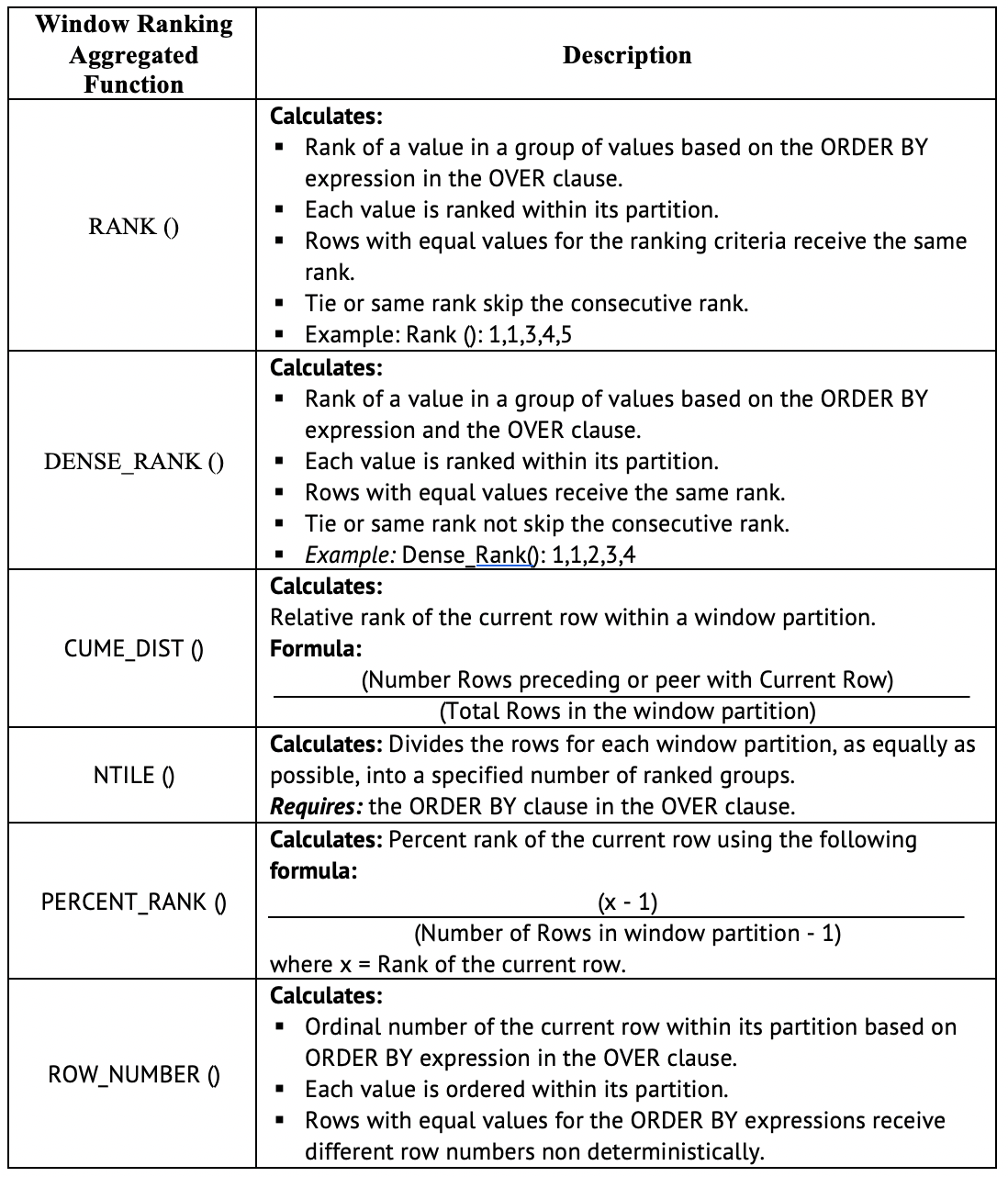Window Ranking Aggregated Function

*3. Window Analytical Function: *Consist one of the supporting ranking function i.e. FIRST_VALUE(), LAST_VALUE(), NTH_VALUE().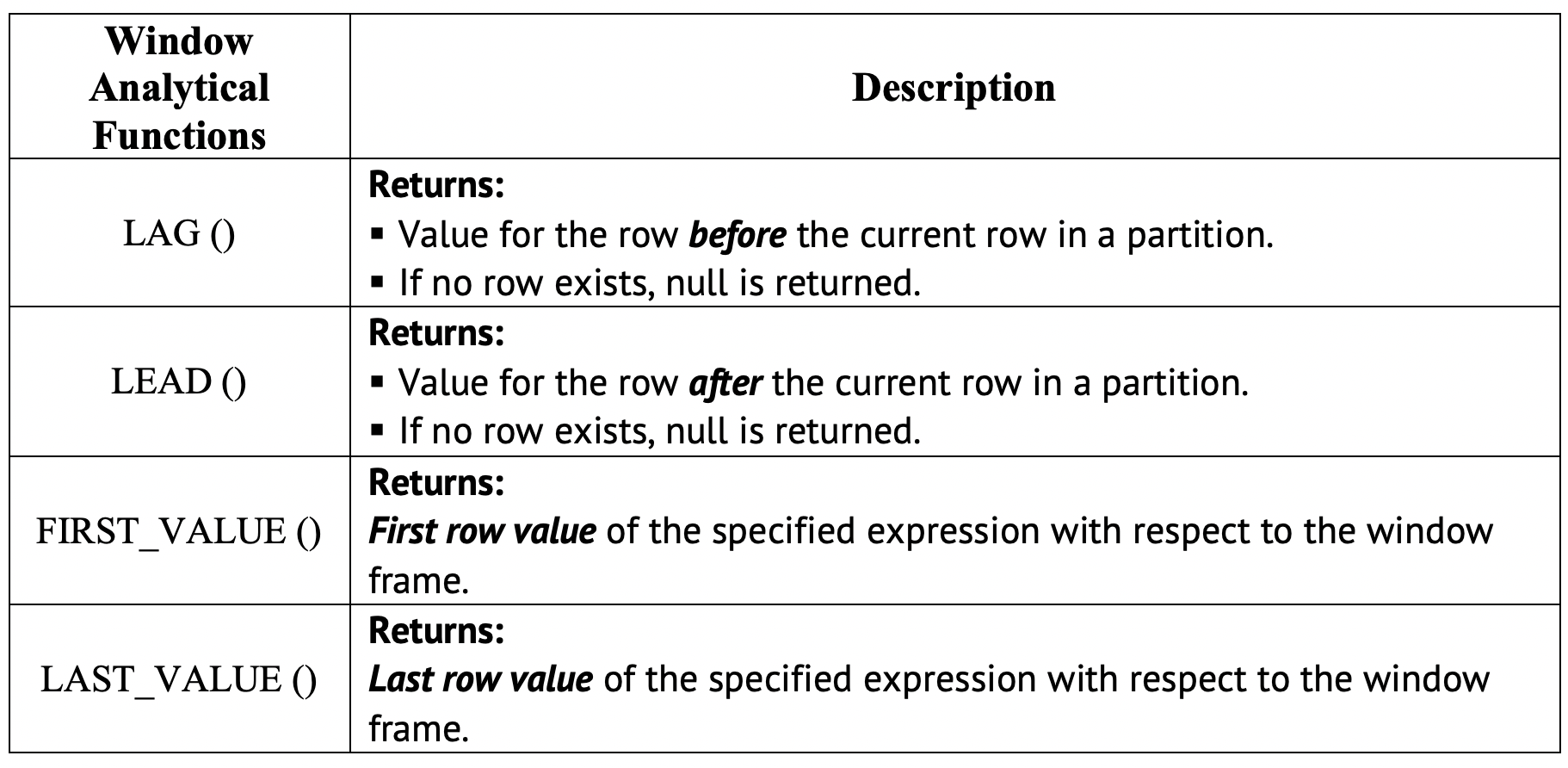Window Analytical Function

Over() clause:

Over() clause is used to define the partitioning and ordering of rows (i.e. a window) for the functions (i.e. avg, count, etc.) to operate on. Hence, called windows function.

Over() clause have the following parameters:

1. Order by: Defines the logical order of the row.

*For example:- *Rank the product_ids with respect to the product price.

``````Query:
Select product_id, price, Rank() OVER (ORDER BY price desc) AS Average_price
From orders;``````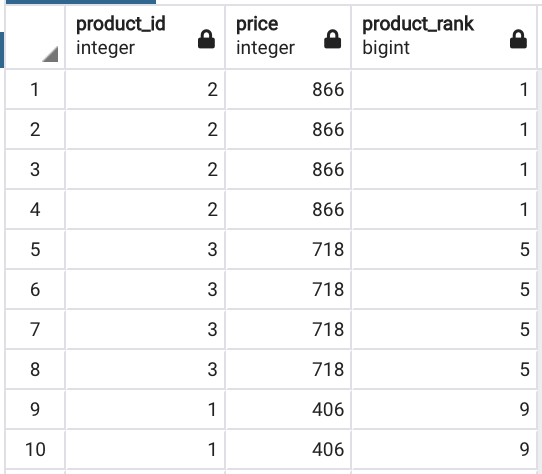Parameter — Order by

*2. Partition by:- *Divides the query result set into _partitions _i.e. window function applied to each partition separately.

*For example:- *Calculate the Average_Order_Price by gender i.e. partition by gender.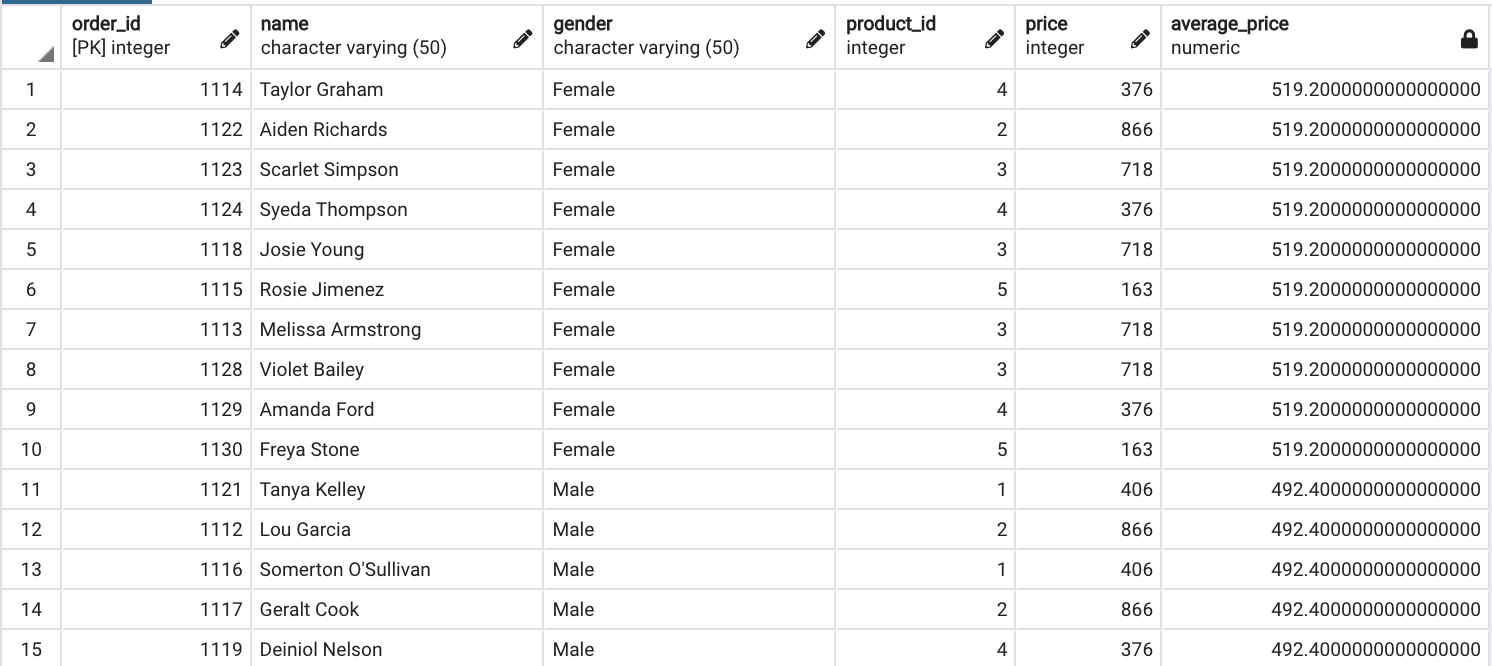Parameter — Partition by

Observation:

The average_price has been calculated by considering partition column i.e. gender. Hence, the same average_price has been calculated for each gender category.

## Introduction to Structured Query Language SQL pdf

SQL stands for Structured Query Language. SQL is a scripting language expected to store, control, and inquiry information put away in social databases. The main manifestation of SQL showed up in 1974, when a gathering in IBM built up the principal model of a social database. The primary business social database was discharged by Relational Software later turning out to be Oracle.

## 15 Machine Learning and Data Science Project Ideas with Datasets

Learning is a new fun in the field of Machine Learning and Data Science. In this article, we’ll be discussing 15 machine learning and data science projects.

## Most popular Data Science and Machine Learning courses — July 2020

Most popular Data Science and Machine Learning courses — August 2020. This list was last updated in August 2020 — and will be updated regularly so as to keep it relevant

## Exploratory Data Analysis is a significant part of Data Science

You will discover Exploratory Data Analysis (EDA), the techniques and tactics that you can use, and why you should be performing EDA on your next problem.

## Why You Should Learn R — Learn Data Science with Dataquest

Why should you learn R programming when you're aiming to learn data science? Here are six reasons why R is the right language for you.